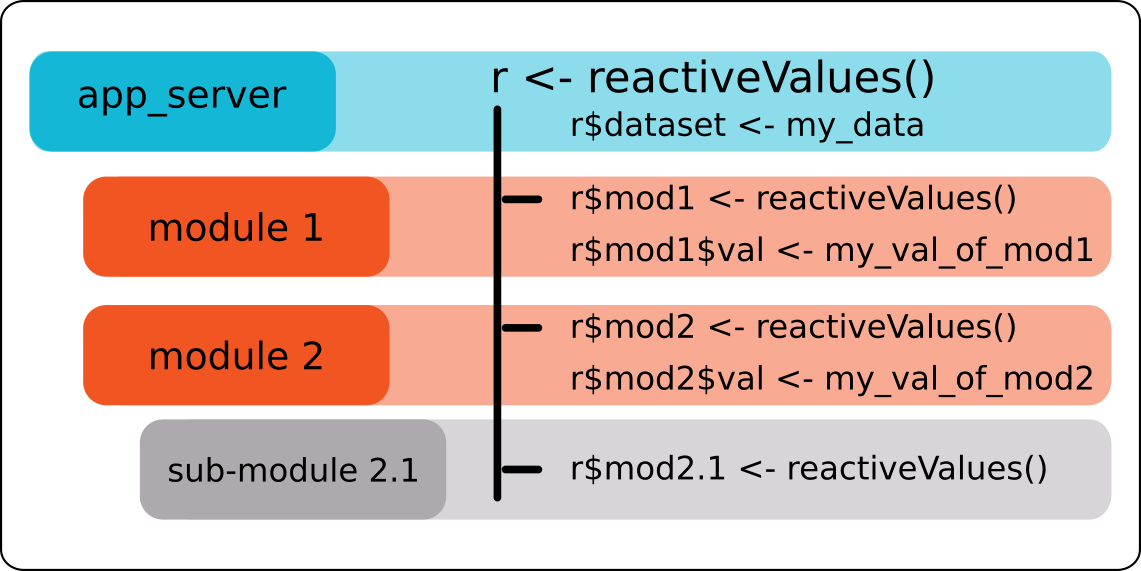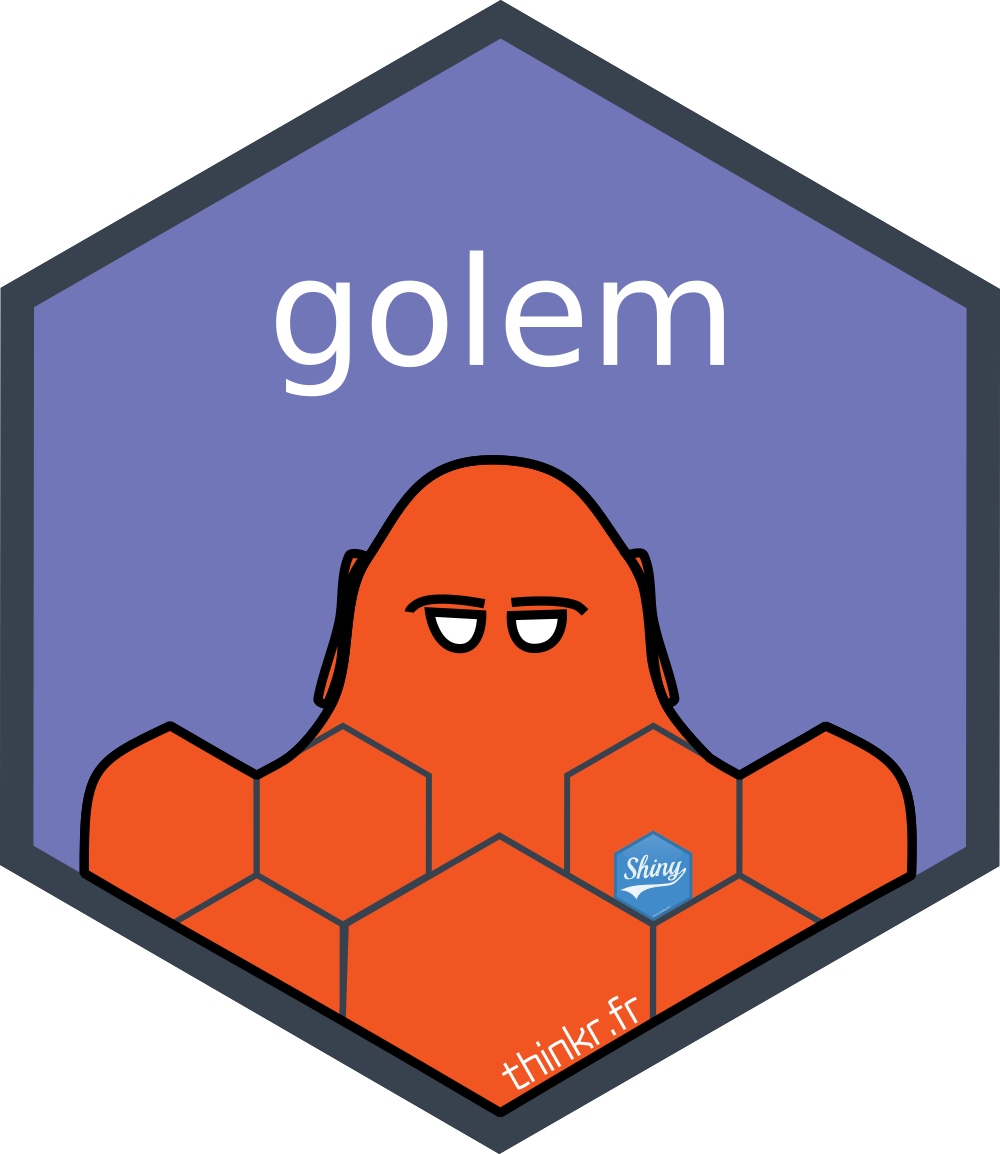# Communication between modules and its whimsTags : shiny
Date :
As part of the development of a Shiny application for production using {golem}, we recommend, among other things, working with Shiny-modules. The communication of data between the different modules can be complex. At ThinkR we use a strategy: the stratégie du petit r. We explain everything in this article.

## What is a module?

A module is the combination of two functions. These functions contain input parameters. As for a function that we could define as pure, what happens inside the module is made to stay there and is not supposed to be in the global environment, except the last object created if it is exported. As with a classic function, a parameter not declared in the function, but which exists in the global environment, can be used in it.
``````a <- 5
my_sum <- function(b) {
b + a
}
my_sum(b = 3)``````
``##  8``
But this is not a reproducible way of working. The function is not independent of the code in which it exists.If you follow ThinkR news, you know that to develop our shiny applications we use package {golem}. {golem} allows development in the form of modules. This makes it easier to maintain the application over time and this encourages group work. As we work with {golem}, we create an R package. By analogy with functions, modules must be able to be considered as independent, documentable and testable with unit tests. This ensures the maintainability of the package. Thus, one could consider that a module could be re-used as is in another Shiny application. This is not always the case, but it is what we recommend to keep in mind when developing modules.

## How to implement the “stratégie du petit r”

The “stratégie du petit r” allows modules to communicate using a global `reactiveValues` that passes through each module to retrieve the different results that we want to share between modules.

#### An example of a Shiny application

Let’s take a reproducible example of an independent shiny application that allows you to select a column from a selected dataset. The selected columns are displayed in a table.
``````library(shiny)
# UI
ui <- fluidPage(
selectInput("dataset", "Choose a dataset:",
choices = c("rock", "pressure", "cars")),
selectInput("colonnes",label = "Choose some columns", choices = NULL, multiple = TRUE),
tableOutput("table")
)
# Server
server <- function(input, output, session) {
datasetInput <- reactive({
switch(input\$dataset,
"rock" = rock,
"pressure" = pressure,
"cars" = cars)
})

observe({
colonnes <- names(datasetInput())
updateSelectInput( session, "colonnes", choices = colonnes)
})

data <- reactive({
req(input\$colonnes)
datasetInput()[, input\$colonnes]
})
output\$table <- renderTable({
})
}
shinyApp(ui, server)``````

#### Transform into a module

For the example, we will pass in module only the part “selection of a column and visualization of its content”. Selection of the dataset is done outside the module. Transforming into a module is realised using [{golem}] (//github.com/ThinkR-open/golem). We will not detail all the steps to use {golem}, you will find more information here and there. We will simply transform the previous application into a module. First step, create a golem for our application:
``golem::create_golem("~/thinkr/appdemo")``
Then we have:
To create a module, use the following function:
``golem::add_module("select_view")``
Then, we have a file `R/mod_select_view.R` created.
``````# Module UI
#' @title   mod_select_view_ui and mod_select_view_server
#' @description  A shiny Module.
#'
#' @param id shiny id
#' @param input internal
#' @param output internal
#' @param session internal
#'
#' @rdname mod_select_view
#'
#' @keywords internal
#' @export
#' @importFrom shiny NS tagList
mod_select_view_ui <- function(id){
ns <- NS(id)
tagList(
)
}
# Module Server
#' @rdname mod_select_view
#' @export
#' @keywords internal
mod_select_view_server <- function(input, output, session){
ns <- session\$ns
}
## To be copied in the UI
# mod_select_view_ui("select_view_ui_1")
## To be copied in the server
# callModule(mod_select_view_server, "select_view_ui_1")``````

#### The “stratégie du petit r” from the main “server” file

Before coding, it is necessary to ensure the availability of data for our future module. In our example, to fully understand the “stratégie du petit r”, we consider the selection of the main dataset outside the column selection module. To do this, we will create a “reactiveValues” that will retrieve the user’s choice. Side “support_ui” for the moment (equivalent to ui.R):
``````app_ui <- function() {
tagList(
# Leave this function for adding external resources
# List the first level UI elements here
fluidPage(
h1("appdemo"),
selectInput("dataset", "Choose a dataset:",
choices = c("rock", "pressure", "cars"))
)
)
}``````
App_server side (equivalent to server.R):
``````app_server <- function(input, output,session) {
# List the first level callModules here
r <- reactiveValues()
observe({
r\$dataset <- switch(input\$dataset,
"rock" = rock,
"pressure" = pressure,
"cars" = cars)
})
}``````
So our dataset is stored in `r\$dataset`. That is the reason for the name: “stratégie du petit r”.

#### The UI side of our module

The UI side of a module is really no different from the “classic” UI. It is not affected by the “stratégie du petit r”.
``````mod_select_view_ui <- function(id){
ns <- NS(id)
tagList(
selectInput(ns("colonnes"),label = "Choose some columns", choices = NULL, multiple = TRUE),
tableOutput(ns("table"))
)
}``````
You must use the `ns` function around the “inputId” and “outputId” to make them unique.

#### The server side of our module

The server side must consider the data available in the global environment and be responsive to any changes in this data. To do this, the `reactiveValues` named `r` is shared with our module. For the documentation of the “server” function, we add a `r` parameter to our module.
``````mod_select_view_server <- function(input, output, session, r){
ns <- session\$ns

observe({
colonnes <- names(r\$dataset)
updateSelectInput( session, "colonnes", choices = colonnes)
})

data <- reactive({
req(input\$colonnes)
r\$dataset[, input\$colonnes]
})
output\$table <- renderTable({
})
}``````
Our `mod_select_view_view_server` function now takes a `r` parameter which will be the “reactiveValues” between modules. Within the Shiny application, you can use the content of `r\$dataset`, i.e. the dataset chosen by the user.

#### Calling the module in the general app

At the general UI level (“app_ui”), it is necessary to add the function `mod_select_view_ui`.
``````app_ui <- function() {
tagList(
# Leave this function for adding external resources
# List the first level UI elements here
fluidPage(
h1("appdemo"),
selectInput("dataset", "Choose a dataset:",
choices = c("rock", "pressure", "cars")),
mod_select_view_ui("select")
)
)
}``````
And on the “app_server” side, the associated `callModule`
``````app_server <- function(input, output,session) {
# List the first level callModules here
r <- reactiveValues()
observe({
r\$dataset <- switch(input\$dataset,
"rock" = rock,
"pressure" = pressure,
"cars" = cars)
})
callModule(mod_select_view_server,id = "select",session = session, r = r)
}``````
All this for this? Is that all? We already know that. Your article is useless, Cervan. we need crunchy! Stay with us reader, the crunchy part is coming!!! We now have an app where the modules can communicate with each other. I will now introduce a problem related to `reactiveValues`, refreshing lists in “reactiveValues”.

## The whims of reactiveValues

In the previous example, we detail how to use the “stratégie du petit r”. That’s when things start to get interesting. Here is a piece of code that has caused some problems in applications:
``````library(shiny)
ui <- fluidPage(
actionButton("pierre",label = "pierre"),
actionButton("henri",label = "henri")
)
server <- function(input, output, session) {
r <- reactiveValues()
observe({
r\$test\$pierre <- input\$pierre
})
observe({
r\$test\$henri <- input\$henri
})
observeEvent(r\$test\$pierre,{
message("Normalement, je clique sur pierre!")
})
}
shinyApp(ui, server)``````
If you click once on “pierre” and only then on “henri”, you will see that the message “Normalement, je clique sur pierre!” is present for each click on “henri” in your console. In other words, the reactivity of a list in a `reactiveValues’ is propagated if one of the items in this list changes. So you can imagine the impact this can have on communication between modules and the possibility of having code restarted several times. With this piece of code, it’s even more obvious:
``````library(shiny)
ui <- fluidPage(
actionButton("pierre",label = "pierre"),
actionButton("henri",label = "henri"),
textOutput("text")
)
server <- function(input, output, session) {
r <- reactiveValues()
observe({
r\$test\$pierre <- input\$pierre
})
observe({
r\$test\$henri <- input\$henri
})
observeEvent(r\$test\$pierre,{
message("Pourtant j'ai cliqué sur henri....")
})
output\$text <- renderText({
time <- Sys.time()
Sys.sleep(5)
paste0(r\$test\$pierre," ",time)
})
}
shinyApp(ui, server)``````
This problem arises for each element at the same sub-list level except for the first one. In other words, here we have no problem with this code :
``````library(shiny)
ui <- fluidPage(
actionButton("pierre",label = "pierre"),
actionButton("henri",label = "henri"),
textOutput("text")
)
server <- function(input, output, session) {
r <- reactiveValues()
observe({
r\$pierre <- input\$pierre
})
observe({
r\$henri <- input\$henri
})
observeEvent(r\$pierre,{
message("Pourtant j'ai cliqué sur henri....")
})
output\$text <- renderText({
time <- Sys.time()
Sys.sleep(5)
paste0(r\$pierre," ",time)
})
}
shinyApp(ui, server)``````
In the first example, the results of our buttons were stored in `r\$test` so we had a list in a `reactiveValues` and that’s the problem. In the second example, we stored directly the buttons at the root of the `reactiveValues`, `r\$pierre` and `r\$henri`. We could then not make a sub-list in a `reactiveValues`. But this is not covenient. If we have several modules that store results in this `reactiveValues`, we won’t be able to remember where they come from. Not really easy for debugging. How to correct this problem which could have significant impacts on communication between modules?

## The “stratégie du petit r”, yes, but with nested reactiveValues

We’ll have to use the “nested” “reactiveValues”! Uh, is that coming out of your hat? Yes. A little bit. But it’s not really complicated.
Since for each first element of a `reactiveValues` the problem does not arise, we will have :
``r <- reactiveValues(test = reactiveValues())``
In this case, the problem is solved and clicking on “henri” does not trigger “pierre”! Example:
``````library(shiny)
ui <- fluidPage(
actionButton("pierre",label = "pierre"),
actionButton("henri",label = "henri"),
textOutput("text")
)
server <- function(input, output, session) {
r <- reactiveValues(test = reactiveValues())
observe({
r\$test\$pierre <- input\$pierre
})
observe({
r\$test\$henri <- input\$henri
})
observeEvent(r\$test\$pierre,{
message("Pourtant j'ai cliqué sur henri....")
})
output\$text <- renderText({
time <- Sys.time()
Sys.sleep(5)
paste0(r\$test\$pierre," ",time)
})
}
shinyApp(ui, server)``````
Voila. That’s it. A big name, for a small modification that changes everything !

We have explored other possibilities for communication between modules, but this is often at the expense of code readability.
• (+) An advantage of this strategy is that the data is not copied several times in the RAM.
• (+) The tree structure between modules is easy to identify et we can easily find where this or that variable comes from.
• (-) A disadvantage is that our module now depends on this `r\$dataset`. If you want to reuse it in another application, you will need a `r\$dataset`. Even if, at ThinkR, we develop Shiny modules as if they should be independent, the reality is that reusable modules represent only a small part of the developed modules. This constraint is therefore not really important. In addition, if the module were to be reused in another application, the associated documentation must indicate this constraint, in the same way that a traditional function forces the user to use a data or parameter format, or to choose input parameters.

## To go further

Want to know more about {golem} and our recommendations around the development of shiny applications:Cervan Girard

Data scientist spécialiste du jonglage avec les serveurs et docker17/01/2022

08/03/2022

08/03/2021

04/03/2021

01/03/2021